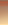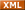# 阿里技术学习博客IT博客 联系 聚合管理
1,在函数中,传递数组时

\$detail=explode("|",\$usertemp);
return \$detail;
}

global \$detail;
\$detail=explode("|",\$usertemp);
}

2,(这个代码用于得到程序目录对应的网址,推荐使用)
\$urlarray=explode('/',\$HTTP_SERVER_VARS['REQUEST_URI']);
\$urlcount=count(\$urlarray);unset(\$urlarray[\$urlcount-1]);
\$ofstarurl='http://'.\$HTTP_SERVER_VARS['HTTP_HOST'].implode('/',\$urlarray);

\$pre_urlarray=explode('/',\$HTTP_SERVER_VARS['HTTP_REFERER']);
\$pre_url=array_pop(\$pre_urlarray);

3,在循环中判断时,数值判断使用恒等要比等于高效
\$a=2;\$b=2;

if(\$a==\$b)\$c=\$a;

if(\$a===\$b)\$c=\$a;

4,mysql 查询时尽量使用where in 少用 limit
limit查多记录的前几条, 速度很快, 但是查询最面几条就会慢

5,NT服务器数据操作稳定性不及unix/linux

6,输出前使用尽量使用 ob_start(); 可以加快输出速度,适用NT或nuli/linux,对unlix类服务器 如果使用 ob_start('ob_gzhandler');输出效率将更高

7,判断的时候尽量使用if(\$a==他的值) 否定的时候尽量使用if(empty(\$a)),因为这样程序运行更快速

8,使用不等时 != 与 <> 效率相当

9,个人经验得 使用 \$a="11111111111111"; 的效率和 \$a='11111111111111'; 相当.并不象书本说的相差很大

10,使用规范的SQL语句, 会有利于MySQL的解析

11,使用

if(\$online){
\$online1=\$online;
}

if(\$online)

12,使用
\$handle=fopen(\$filename,wb);
flock(\$handle,LOCK_SH);
fclose(\$handle);

file(\$filename);

13,截断字符串优化函数(可避免?字符出现)
function substrs(\$content,\$length) {
if(strlen(\$content)>\$length){
\$num=0;
for(\$i=0;\$i<\$length-3;\$i++) {
if(ord(\$content[\$i])>127)\$num++;
}
\$num%2==1 ? \$content=substr(\$content,0,\$length-4)content=substr(\$content,0,\$length-3);
\$content.=' ...';
}
return \$content;
}

14,程序中屏蔽大小写
for (\$asc=65;\$asc<=90;\$asc++)
{ //strtolower() 此函数在一些服务器会产生乱码!
if (strrpos(\$regname,chr(\$asc))!==false)
{
\$error="为了避免用户名混乱,用户名中禁止使用大写字母，请使用小写字母";
\$reg_check=0;
}

15,不使用 file();和不使用 fget();(不稳定或速度慢) 取一数组函数

function openfile(\$filename,\$method="rb")
{
[email=\$handle=@fopen(\$filename,\$method]\$handle=@fopen(\$filename,\$method[/email]);
@flock(\$handle,LOCK_SH);
@fclose(\$handle);
\$filedata=str_replace("\n","\n<ofstar:>",\$filedata);
\$filedb=explode("<ofstar:>",\$filedata);
//array_pop(\$filedb);
\$count=count(\$filedb);
if(\$filedb[\$count-1]==''){unset(\$filedb[\$count-1]);}
return \$filedb;
}
//这个函数虽然代码比较多,不过在速度和稳定性上优势很大!

PHP应用程序的性能优化

by soola

<?php
include_once("Benchmark/Timer.php");
\$bench = new Benchmark_Timer; \$bench->start();
\$bench->setMarker('Start of the script'); 现在处于睡眠状态几分钟
sleep(5); \$bench->stop(); // 从计时器中获得分析信息
print_r(\$bench->getProfiling());
?>

Array
(
 => Array
(
[name] => Start
[time] => 1013214253.05751200
[diff] => -
[total] => 0
)
 => Array
(
[name] => Start of the script
[time] => 1013214253.05761100
[diff] => 9.8943710327148E-05
[total] => 9.8943710327148E-05
)
 => Array
(
[name] => Stop
[time] => 1013214258.04920700
[diff] => 4.9915959835052
[total] => 4.9916949272156
)
)

\$bench->start()、\$bench->setMarker()和\$bench->stop()，与这些表目有关的数字是相当简单的，现在我们来仔细地研究这些数字：

 => Array
(
[name] => Start
[time] => 1013214253.05751200
[diff] => -
[total] => 0
)

time表目指的是何时对Benchmark_Timer()的start()方法调用的UNIX的timestamp，diff表目表示这次调用和上次调用之间的时间间隔，由于这里没有上一次，因此显示出了一个破折号，total表目指的是自测试开始到这一特定的调用之前代码运行的总的时间。下面我们来看看下一个数组的输出：

 => Array
(
[name] => Start of the script
[time] => 1013214253.05761100
[diff] => 9.8943710327148E-05
[total] => 9.8943710327148E-05
)

>0.0000989秒）后开始调用\$bench->setMarker(....)。

PEAR::Benchmark库中的另一个被称作Benchmark_Iterator的类能够很好地解决这一个问题，它能够针对特定的函数或类的方法，显示其分析信息。它的用途是能够能够从测试中获得一致的结果，因为我们知道，如果运行一段脚本一次，其运行时间为10秒，并不意味着它每次的运行时间总是10秒。

In any case, let's see some examples:

// 连接数据库的代码

include_once("DB.php");
\$dsn = array(
'phptype' => 'mysql',
'hostspec' => 'localhost',
'database' => 'database_name',
);
\$dbh = DB::connect(\$dsn); function getCreatedDate(\$id)
{
global \$dbh; >\$stmt = "SELECT created_date FROM users WHERE id=\$id";
// 在这里使用PEAR:B
\$created_date = \$dbh->getOne(\$stmt);
if ((PEAR::isError(\$created_date)) ||
(empty(\$created_date))) {
return false;
} else {
return \$created_date;
}
}include_once 'Benchmark/Iterate.php';
\$bench = new Benchmark_Iterate; // 运行getDate函数10次
\$bench->run(10, 'getCreatedDate', 1);// 打印分析信息
print_r(\$bench->get());
?>

Array
(
 => 0.055413007736206
 => 0.0012860298156738
 => 0.0010279417037964
 => 0.00093603134155273
 => 0.00094103813171387
 => 0.00092899799346924
 => 0.0010659694671631
 => 0.00096404552459717
 => 0.0010690689086914
 => 0.00093603134155273
[mean] => 0.0064568161964417
[iterations] => 10
)

http://ming1212.bokee.com/1393104.html，大家如果有兴趣，请到作者的博客看相应的技术文章。
posted on 2007-04-20 14:51 阿里爸爸 阅读(74) 评论(0)  编辑 收藏 引用